# Introduction to Autoencoders

## Introduction to Autoencoders

To find hidden connections between data and represent data in a more compact dimension, autoencoders are neural network-based models that are used for unsupervised learning. To train a neural network model, the autoencoders transform unsupervised learning issues into supervised learning problems. Only the input is sent to the output. An encoder network compresses the input into a smaller encoded representation, while a decoder network decodes the encoding to reconstruct the input.

The lower-dimensional representation of the data and various intriguing complicated linkages between the data are displayed in the encoding created by the encoder layer.

##### Components of Autoencoders

The components of an autoencoder are as follows:

• Encoder: The encoder is the component of the network that receives input and generates a lower-quality signal.
• Bottleneck: The lower-dimensional hidden layer is the source of the encoding. The number of nodes in the bottleneck layer is reduced, and it also determines how the input is encoded in terms of dimension.
• Decoder: The decoder receives the input and reconstructs it using the encoding.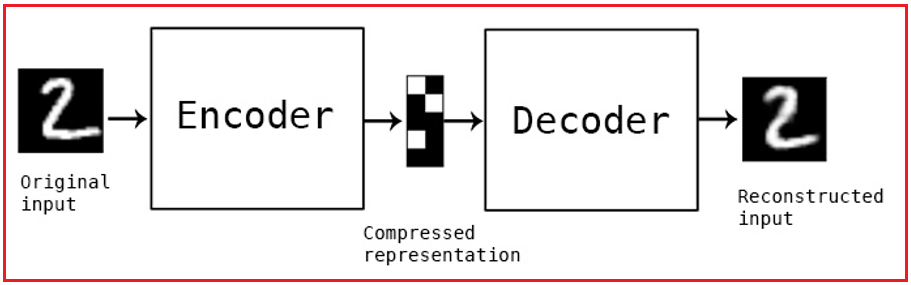In a perfect autoencoder model, these factors are balanced:

1. It is sensitive enough to the inputs to precisely generate a reconstruction.
2. Insensitive enough to the inputs such that the model does not simply memorize or overfit the training data.

This trade-off forces the model to discard redundant input and keep just the variations in the data necessary to rebuild the input.

#### Autoencoder Applications

Some of the applications for autoencoders include:

The main application of autoencoders is anomaly detection: Autoencoders, as we all know, produce encodings that essentially record the relationships between data. The encoder and decoder parameters will now be trained to best depict the relationships on the datasets if we train our autoencoder on a specific dataset. Consequently, will be able to replicate any provided data from that type of dataset in the best possible manner. So, the reconstruction error is lower if data from that specific dataset is transmitted through the autoencoder. However, sending a different type of data via the autoencoder will result in a significant reconstruction mistake. Applying the right cutoff will allow us to produce an anomaly.

Noise Removal: The use of autoencoders for noise reduction can be facilitated by providing noisy data as input and clean data as output, and then training the autoencoder on these given data pairs. This is because noise points typically lack correlation. Since autoencoders must represent the data in the most diminutive possible dimensions, the encodings now usually contain just the most significant relationships, discarding the random ones. Therefore, the decoded data that is produced as an autoencoder’s output does not contain all additional relations and noise.

Auto Encoders as Generative Models: Before the development of GANs, autoencoders were employed as generative models. Variational autoencoders are one of the modified methods of autoencoders that are applied to generative tasks.

Using autoencoders for collaborative filtering: Matrix factorization techniques are typically used in collaborative filtering, however, autoencoders can learn the dependencies and forecast the item-user matrix.

#### Understanding Autoencoders

To get a better understanding of how autoencoders work, let us look into some of the use cases:

##### 1. Data Compression –

Autoencoders are frequently employed in data compression and compressed data transfer. Here, I’ll give a brief explanation of how autoencoders function to give some fundamental context for when data compression with them might be applied. A class of neural networks called autoencoder functions in a self-supervised manner.

Encoder, decoder, and coder or latent space are the three fundamental components of autoencoders. Thus, as is customary, you provide the encoder with the data you wish to utilize, and it either encodes it or just extracts its valuable components and stores them in latent space. Then the decoder repeats the process but in the other direction.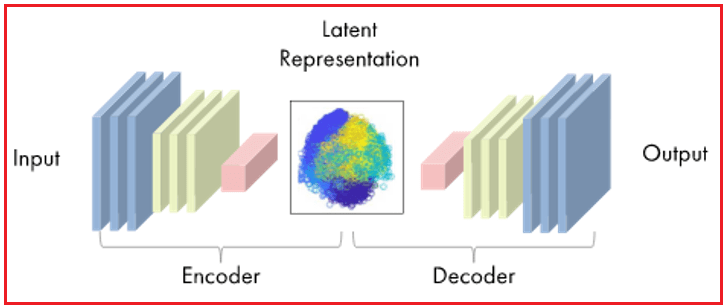One of the key benefits of autoencoders is that data compression can be used to solve problems with data transmission. Depending on your request, picture a server uploading a Latent Space Representation of the input image to the internet exclusively.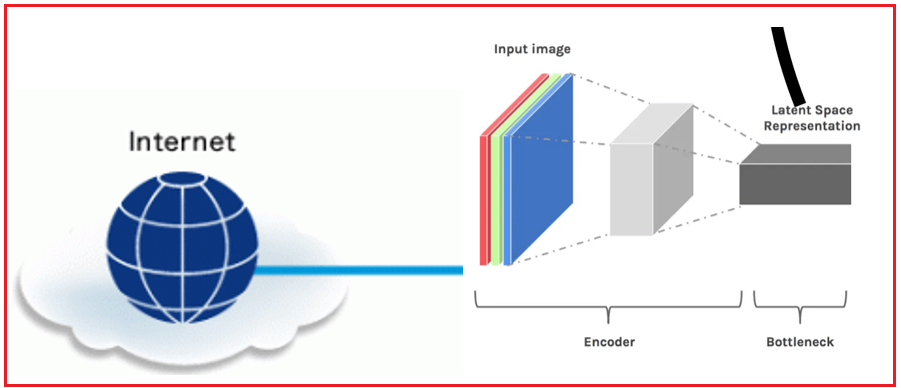The Latent Space Representation is then downloaded in the same way to your mobile device, where it is then decoded to produce output results (such as images, videos, or audio files) with even higher resolution or quality than the input: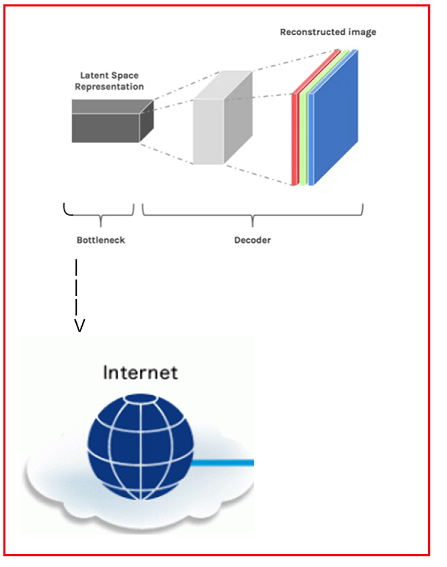##### 2. Data Denoising –

One of the biggest issues in data transmission is the quality loss of the data (images, audio, and video). Denoising data is a challenge since data, including images and audio, might lose quality due to bandwidth or connectivity issues. One of the cool benefits of autoencoders is data denoising.

In a perfect world, our autoencoder would be sensitive enough to reconstruct our latent space representation and insensitive enough to encode input data broadly. One technique to do this is to introduce random noise to the input data to corrupt it and then encode the resulting corrupted data. After that, the output of the decoder is compared to the original image.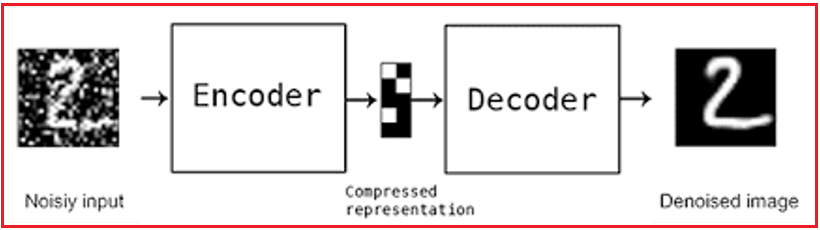So how exactly does an autoencoder operate? It involves only a few easy steps:

The input (x) is received by the encoder, which maps it to a vector (z), the latent space; the vector (z), the latent space, is then received by the decoder, which creates a reconstructed input (x~).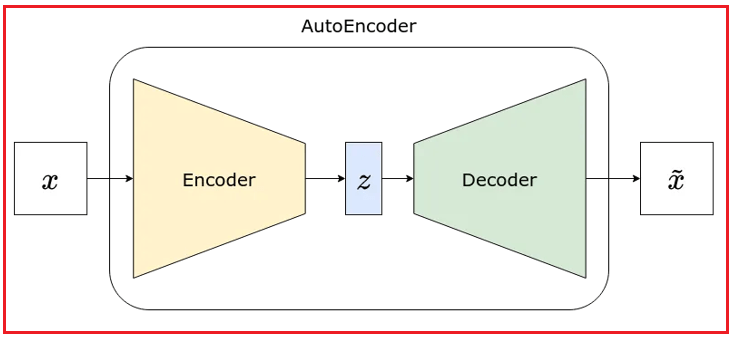#### Building an Autoencoder Model

Here is a step-by-step guide for the same –

##### 1. Load the data and import the required libraries –

The first step is to load the data and import the required libraries. We’ll utilize the MNIST collection of handwritten digits for this example.

```import tensorflow as tf
from tensorflow.keras.datasets import mnist

(x_train, _), (x_test, _) = mnist.load_data()
```
##### 2. Data preprocessing –

The data will be then pre-processed by scaling the pixel values to range between 0 and 1.

```x_train = x_train.astype('float32') / 255.
x_test = x_test.astype('float32') / 255.

x_train = x_train.reshape((len(x_train), 28, 28, 1))
x_test = x_test.reshape((len(x_test), 28, 28, 1))
```
##### 3. Define the Architecture of the Autoencoder –

We will discuss the autoencoder’s architecture. In this example, we will utilize a straightforward design with just one layer each for encoding and decoding.

```# Encoder
encoder_input = tf.keras.Input(shape=(28, 28, 1))
x = tf.keras.layers.Conv2D(16, 3, activation='relu', padding='same')(encoder_input)
x = tf.keras.layers.Conv2D(8, 3, activation='relu', padding='same')(x)
x = tf.keras.layers.Conv2D(8, 3, activation='relu', padding='same')(x)

# Decoder
x = tf.keras.layers.Conv2D(8, 3, activation='relu', padding='same')(encoder_output)
x = tf.keras.layers.UpSampling2D(2)(x)
x = tf.keras.layers.Conv2D(8, 3, activation='relu', padding='same')(x)
x = tf.keras.layers.UpSampling2D(2)(x)
x = tf.keras.layers.Conv2D(16, 3, activation='relu')(x)
x = tf.keras.layers.UpSampling2D(2)(x)
decoder_output = tf.keras.layers.Conv2D(1, 3, activation='sigmoid', padding='same')(x)

autoencoder = tf.keras.Model(encoder_input, decoder_output)
autoencoder.summary()
```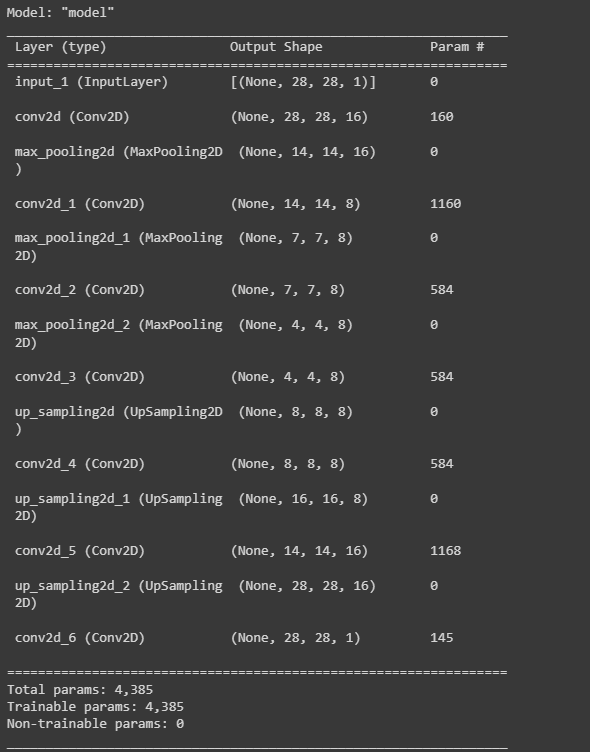##### 4. Create the model –

The loss function and optimizer will be specified as we compile the autoencoder model.

`autoencoder.compile(optimizer='adam', loss='binary_crossentropy')`
##### 5. Model Compilation –

On the training set of data, we’ll train the autoencoder model.

```autoencoder.fit(x_train, x_train,
epochs=10,
batch_size=128,
shuffle=True,
validation_data=(x_test, x_test))
```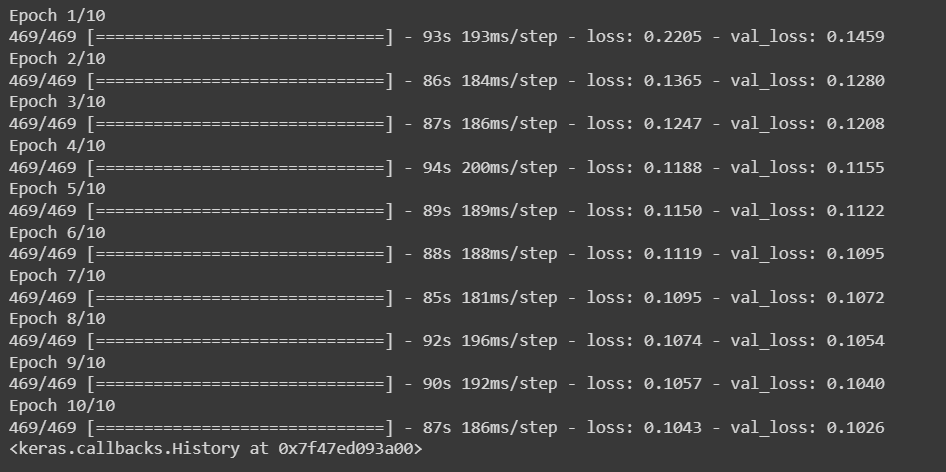##### 6. Model Evaluation –

We will then assess the autoencoder model using the test data.

```score = autoencoder.evaluate(x_test, x_test, verbose=0)
print('Test loss:', score)
```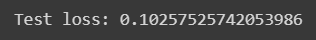That is all, then! An autoencoder model that can compress and decompress pictures is now available. Naturally, you can alter the architecture and hyperparameters to meet your unique requirements.